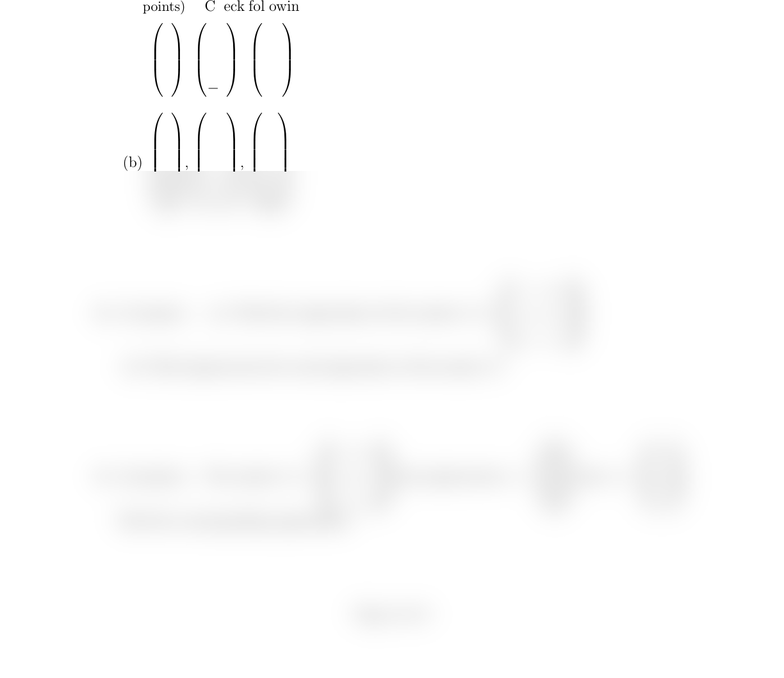# MATH51 PastExams Exam3-S11

26 views2 pagesMath 38 Spring 2011
Midterm Exam 3
Instructions. No calculators, notes, or books are allowed. Please make sure all electronic
devices are turned oﬀ and out of sight. Show all work and cross out work you do not want
have neither given nor received assistance on this exam.
Good luck!
1. (10 points): Check following sets of vectors for independence (NO PARTIAL CREDIT):
(a)
1
7
2
,
2
4
1
,
1
0
1
,
2
0
1
(b)
4
3
2
1
,
2
2
4
8
,
6
8
8
10
2. (15 points): (a) Find the eigenvalues of the matrix A=
1 0 0
1 2 0
0 1 3
(b) Find eigenvectors for each eigenvalue of the matrix A.
3. (10 points): The matrix A=
1 1 0
1 0 1
1 1 0
has eigenvectors v=
1
1
1
and w=
1
2
1
.
Find the corresponding eigenvalues.
Page 1 of 2
Unlock document

This preview shows half of the first page of the document.
Unlock all 2 pages and 3 million more documents.

## Document Summary

Please make sure all electronic devices are turned o and out of sight. Show all work and cross out work you do not want graded! With your signature you are pledging that you have neither given nor received assistance on this exam. Good luck: (10 points): check following sets of vectors for independence (no partial credit): 10: (15 points): (a) find the eigenvalues of the matrix a = 1 3 (b) find eigenvectors for each eigenvalue of the matrix a: (10 points): the matrix a = Page 1 of 2: (10 points): use the row reduction method to solve the following systems: x1 + 2x2 + x3 x4 x5 = 2. 2x1 + 2x2 + 2x3 3x4 2x5 = 1. X3 + 2x4 + x5 = 1. No credit for any other method: (20 points): solve the system of linear di erential equations d~x = a~x, where.